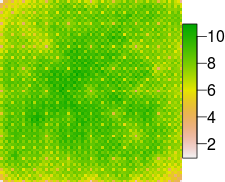# Random fractal maps

I wanted to share the results of my quest to produce random fractal maps. I eventually went with the GRASS r.surf.fractal script but before that I recreated the 2D midpoint displacement algorithm from Saupe (1988). The results are below. I think its solid but there still appears to be some artifacts which I have yet to track down.``````    #MidPointFM2D####
#X: doubly indexed array of size (N+1)^2
#maxlevel: maximal number of recursions
#sigma: initial standard deviation
#H: Hurst exponent (determines fractal dimension)
#seed seed value for random numbers gen.

library(Oarray)
library(raster)

reps=10

#interpolates inner points
f4<-function(delta,x0,x1,x2,x3){
return((x0+x1+x2+x3)/4+rnorm(1,1,delta))
}
#interpolates edge points
f3<-function(delta,x0,x1,x2){
return((x0+x1+x2)/3+rnorm(1,1,delta))
}

#inputs
6->maxlevel
1->sigma
0.4->H
#seed<-101

#for(kk in 1:reps){
N<-2^maxlevel
X<-Oarray(NA,dim=c(N+1,N+1),offset=0)
#set initial random corners
delta<-sigma
#print(delta)

X[0,0]<-rnorm(1,1,delta)
X[0,N]<-rnorm(1,1,delta)
X[N,0]<-rnorm(1,1,delta)
X[N,N]<-rnorm(1,1,delta)
D<-N
d<-N/2

for(stage in 1:maxlevel){
delta<-delta*(0.5^(0.5*H))#going from grid type I to grid type II
for(x in seq(from=d,to=(N-d),by=D)){#generate mid points;interpolate and offset points
for(y in seq(from=d,to=(N-d),by=D)){
X[x,y]<-f4(delta,X[x+d,y+d],X[x+d,y-d],X[x-d,y+d],X[x-d,y-d])
}
}
#going from grid type II to type I
delta<-delta*(0.5^(0.5*H))
#interpolate and offset boundary grid points
for(x in seq(from=d,to=(N-d),by=D)){
X[x,0]<-f3(delta,X[x+d,0],X[x-d,0],X[x,d])
X[x,N]<-f3(delta,X[x+d,N],X[x-d,N],X[x,N-d])
X[0,x]<-f3(delta,X[0,x+d],X[0,x-d],X[d,x])
X[N,x]<-f3(delta,X[N,x+d],X[N,x-d],X[N-d,x])
}

#interpolate and offset interior grid points

if(stage==2){
for(x in seq(from=d,to=(N-d),by=D)){
for(y in seq(from=D,to=(D),by=D)){
X[x,y]<-f4(delta,X[y+d,x],X[x,y-d],X[x+d,y],X[y,x-d])#try moving x input to y input and vice versa on first and third x,y term
}
}

for(y in seq(from=d,to=(N-d),by=D)){
for(x in seq(from=D,to=(D),by=D)){
X[x,y]<-f4(delta,X[x,y+d],X[x,y-d],X[x+d,y],X[x-d,y])
}
}
}

if(D<(N-d)){
for(x in seq(from=d,to=(N-d),by=D)){
for(y in seq(from=D,to=(N-d),by=D)){
X[x,y]<-f4(delta,X[y+d,x],X[x,y-d],X[x+d,y],X[y,x-d])#try moving x input to y input and vice versa on first and third x,y term
}
}

for(x in seq(from=D,to=(N-d),by=D)){
for(y in seq(from=d,to=(N-d),by=D)){
X[x,y]<-f4(delta,X[x,y+d],X[x,y-d],X[x+d,y],X[x-d,y])
}
}
}
D<-D/2
d<-d/2
#print((sum(is.na(X))/length(X))*100)
outarray<-array(X,dim=c(N+1,N+1))
}
plot(raster(outarray))
``````

### Reference

Saupe, Dietmar. “Algorithms for random fractals.” The science of fractal images. Springer New York, 1988. 71-136.